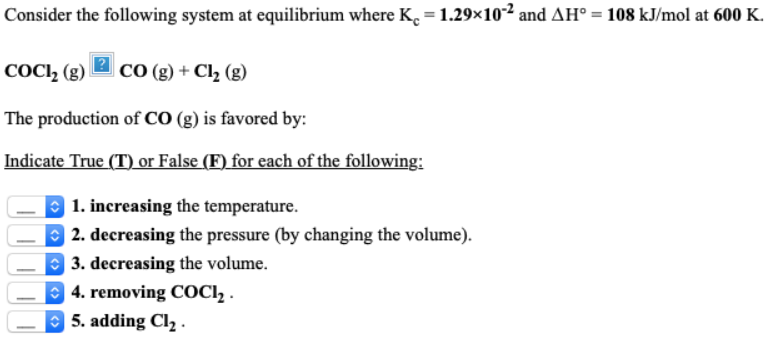# Consider the following system at equilibrium where Kc = 1.29 x 10^-2 and ΔH° = 108 kJ/mol at 600 K. COCl2 (g) -> CO(g) + Cl2(g) The production of CO(g) is favored by: Indicate True (T) or False (F) for each of the following: 1. increasing the temperature. 2. decreasing the pressure (by changing the volume). 3. decreasing the volume. 4. removing COCl2. 5. adding Cl2.